Next: Analyzing the adaptive controller Up: number11 Previous: The importance of being

The controller equations

Recall from Closing the loop'' (FD XVIII No. 5) the equation for a proportional-integral-derivative (PID) controller is: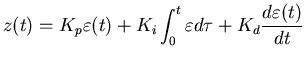(4)

where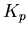is the proportional gain,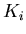is the integral gain and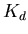is the differential gain.

The quantity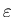is an error signal that is the difference between the commanded input,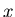and the output of the controlled system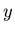. In our earlier investigations we were not much concerned with the internals of the controlled system (often called the plant), all we needed was the output signal. Figure 1 shows a generic schematic of our controller and plant.

For an adaptive system, we need to have an additional equation that describes how the plant behaves so that we can properly adjust the parameters of the controller. So now we have two equations to consider, the controller and the plant. The design of the adaptiveness depends upon the form of both equations. For our example plant, we will use the second order differential equation: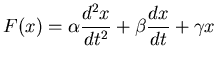(5)

this is a pretty generic model, as a example this can be thought of as a damped mass-spring system where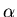is the mass,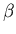is the friction and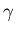is the spring constant.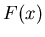would represent the imposed external forces on the system (the input), and the solution to the equation would give the plants response to it.Next: Analyzing the adaptive controller Up: number11 Previous: The importance of being
Skip Carter 2008-08-20